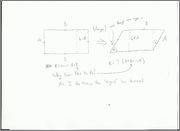Hi there! My question involves the area of a parallelogram. Now, I know how to prove the commonly used formula (b*h) very easily, however, there is a formula given on Wikipedia as an alternative that states....Given two sides B and C with angle (theta), B*C*sin(theta)=Area of a parallelogram. Now the problem is that there is no proof given for this problem and I keep coming up with contradictions in my head. For example, say you start with a rectangle with sides A and B where A does not equal B. Now, take that rectangle and move two of it's angles that are collinear(lie on the same side or line) through an equivalent angle (theta). What you will be left with is a parallelogram whose sides are the same length as the rectangle we started with (A and B). We know that the rectangle has an area of A*B, so it should be safe to assume that the parallelogram has the same area. This is where the trouble comes in (in my head at least). The definition given in Wikipedia states that the area of a parallelogram with two sides A and B and an angle theta is equal to A*B*sin(theta). So, my questions to you is, where is my sin(theta) missing? What mistake(s) have I made? Have there been any incorrect assumptions I have made? Does anyone know a proof for the formula given on Wikipedia? For all those who help me in understanding and using there time to help me out, thank you in advance!

(Image below to describe my reasoning.)Last edited:

why ? "...so it should be safe to assume that the parallelogram has the same area."? If the angle were 90 degrees, the parallelogram would have zero error.

why ? "...so it should be safe to assume that the parallelogram has the same area."? If the angle were 90 degrees, the parallelogram would have zero error.

Yes. But what I am saying is that the angle is less than 90 degrees. All I have done is taken the original rectangle and "Pushed it" so that it went "sideways." I am just wondering why the area would not simply be A*B instead of A*B*sin(theta) because if it were A*B and the angle is less than 90, then the area has decreased where my logical reasoning says it shouldn't have. I haven't done nothing to the original rectangle but "transform" it. The area should remain the same regardless.

If you know the proof to the formula, could you post it as that may clear up some misconceptions on my part. Thank you for your help!

LeonhardEuler
Gold Member
Changing the angle does change the area. Imagine you kept collapsing the angle so it went to 0. You would be left with a line segment with 0 area, just like the formula ABsin(0) would give you.

I suggest you will get valuable insight from drawing your rectangle, upright, and distorted, on graph paper, and count the little squares in both cases.

HallsofIvy
Homework Helper
You seem to be assuming that the area should be the same no matter what the angle is. To see that this is not true, imagine continuing to tilt the side more and more so that angle becomes almost 0. As that side tilts more and more the area becomes smaller and smaller eventually (as the titling side merges the with the adjacent side) going to 0.

(I now see that this is what LeonardEuler said first.)

If you have side A and base B, and theta the angle between them, then the height is just A sin(theta), which gives your base times height. How's that for your proof?

You seem to be assuming that the area should be the same no matter what the angle is. To see that this is not true, imagine continuing to tilt the side more and more so that angle becomes almost 0. As that side tilts more and more the area becomes smaller and smaller eventually (as the titling side merges the with the adjacent side) going to 0.

(I now see that this is what LeonardEuler said first.)

HOLY CRAP! Thank you so much. I cannot believe I didn't think of that! It makes so much sense. It doesn't seem like it when you think about it though...You would think that the area remains constant...GEEZ! Math is exciting! Thank you all and especially you! Very simplistic solution! USE LIMITS! HAHA!

Changing the angle does change the area. Imagine you kept collapsing the angle so it went to 0. You would be left with a line segment with 0 area, just like the formula ABsin(0) would give you.

Sorry. Just realized you said this first! Take the comment I made to "HallsofIvy" and apply it to yourself as well! Thank you!

If you have side A and base B, and theta the angle between them, then the height is just A sin(theta), which gives your base times height. How's that for your proof?

Thank you! I clearly do not think in a direct mannerism...Anyone else left-handed? Thanks again to everyone for your help! I always seem to have problems with the simple math problems, yet I find set theory and linear algebra rather straightforward...